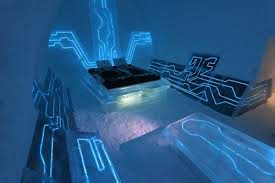Published on Sep 03, 2023

### Introduction

Clustering is one of the important streams in data mining useful for discovering groups and identifying interesting distributions in the underlying data.

This project aims in analyzing and comparing the partitional and hierarchical clustering algorithms namely DBSCAN and k-means (partitional) with Agglomerative and CURE (hierarchical).

The comparison is done based on the extent to which each of these algorithms identify the clusters, their pros and cons and the timing that each algorithm takes to identify the clusters present in the dataset.

Among each clustering algorithm, computation time was measures as the size of data set increased. This was used to test the scalability of the algorithm and if it could be disintegrated and executed concurrently on several machines.

k-means is a partitional clustering technique that helps to identify k clusters from a given set of n data points in d-dimensional space. It starts with k random centers and a single cluster, and refines it at each step arriving to k clusters. Currently, the time complexity for implementing k - means is O (I * k * d * n), where I is the number of iterations. If we could use the KD-Tree data structure in the implementation, it can further reduce the complexity to O (I * k * d * log (n)).

DBSCAN discovers clusters of arbitrary shape relying on a density based notion of clusters. Given eps as the input parameter, unlike k-means clustering, it tries to find out all possible clusters by classifying each point as core, border or noise.

DBSCAN can be expensive as computation of nearest neighbors requires computing all pair wise proximities. Additional implementation includes KD-Trees to store the data which would allow efficient retrieval of data and bring down the time complexity from O(m^2) to O(m log m).

Agglomerative Hierarchical Clustering is one of the non-parametric approaches to Clustering which is based on the measures of the dissimilarities among the current cluster set in each iteration. In general we will start with the points as individual clusters and at each step merge the closest pair of clusters by defining a notion of cluster proximity.

We will implement three algorithms, namely, Single-Linkage Clustering and Complete-Linkage Clustering. We will be analyzing the advantages and drawbacks of Agglomerative Hierarchical Clustering by comparing it with the other Algorithms CURE, DBSCAN and K-Means.

CURE clustering algorithm helps in attaining scalability for clustering in large databases without sacrificing quality of the generated clusters. The algorithm uses KD-Trees and Min Heaps for efficient data analysis and repetitive clustering. The random sampling, partitioning of clusters and two pass merging helps in scaling the algorithm for large datasets. Our implementation would provide a comparative study of CURE against other partitioning and hierarchical algorithm.

### Observations regarding DBSCAN Issues

The following are our observations:

DBSCAN algorithm performs efficiently for low dimensional data.

The algorithm is robust towards outliers and noise points

Using KD Tree improves the efficiency over traditional DBSCAN algorithm

DBSCAN is highly sensitive to user parameters MinPts and Eps. Slight change in the values may produce different clustering results and prior knowledge about these values cannot be inferred that easily.

The dataset cannot be sampled as sampling would affect the density measures.

The Algorithm is not partitionable for multi-processor systems.

DBSCAN fails to identify clusters if density varies and if the dataset is too sparse.